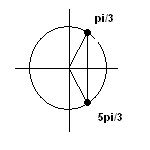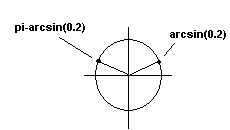# Solve Trigonometric Equations

Tutorial, with detailed explanations, on how to solve trigonometric equations using different methods and strategies and the properties of trigonometric functions and identities. The unit circle helps in locating the solutions once you have the reference angle.
Many more examples on how to solve trigonomteric equations with detailed solutions - Grade 12, are presented in this site.

## Examples with Solutions

### Example 1

Solve the trigonometric equation.(find all solutions)
2 cos x + 2 = 3

Solution to example 1

• solve for cos(x)
cos x = 1/2
• solve for x by finding all values in the interval [0 , 2pi) that satisfy the above trigonometric equation. In this case, with cosine positive and equal to 1 / 2, there are two values: one in the first quadrant of the unit circle.
x1 = pi / 3
• and a second one in the fourth quadrant (see the two solutions in unit circle in figure below).
x2 = 2*pi - pi / 3 = 5*pi / 3• find all solutions using the fact that of cos x has a period of 2pi
x1 = pi / 3 + 2*k*pi
x2 = 5*pi / 3 + 2*k*pi
where k is any integer

conclusion: There is an infinite number of solutions which can be generated by giving different values to k.

### Example 2

Find the solutions in the interval [0 , 2pi) for the trigonometric equation
-5 cos 2x + 9 sin x = -3

Solution to example 2

• change cos 2x to 1 - sin 2x
-5(1 - sin 2x) + 9 sin x = -3
• multiply factors and group to obtain
5 sin2x + 9 sin x -2 = 0
• let u = sinx and substitute to obtain an quadratic equation.
5 u2 + 9 u - 2 = 0
• use any method to solve for u. By the quadratic formula, we obtain two solutions u1 and u2
u1 = [ -9 - sqrt(121) ] / 10 = 1 / 5 = -2
• and
u2 = [ -9 + sqrt(121) ] / 10 = 0.2
• we now solve the equation for x
u1 = sin x = -2
• the above equation has no solutions for x since -2 is not in the range of values of sin(x);
-1 <= sin x <= 1.

u2 = sin x = 0.2
• sin x is positive in the first and second quadrants of the unit circle. There are two solutions to the above equation in [0 , 2 pi)(see unit circle below)x1 = arcsin 0.2
• arcsin 0.2 is the inverse sine function and it can be approximated using a calculator)
x2 = pi - arcsin 0.2
conclusion: There are two solutions to the given equation.

### Example 3

Find all solutions for the trigonometric equation
cot x cos 2x = cot x

Solution to example 3

• subtract cot x from both sides of the equation and simplify
cot x cos 2x - cot x = 0
• Factor cot x
cot x (cos 2x - 1) = 0
• Setting each factor in the above trigonometric equation to zero, we obtain two equations.
cot x = 0 and cos 2x - 1 = 0
• The solutions to equation cot x = 0 are given by
x = pi / 2 + k*pi , k is am integer.
• Equation cos 2x - 1 = 0 gives
cos x = 1 and cos x = -1
• The solutions to the above equations are given by
x = 2k*pi and x = (2k + 1)*pi where k is an integer
• HOWEVER the above cannot be solution to the given equation since cot x is undefined for x = 2k*pi and x = (2k + 1)*pi.
conclusion: The solutions to the given equation are.
x = pi / 2 + k*pi where k is an integer.

Note that many of the techniques used in solving algebraic equations are also used to solve trigonometric equations.